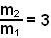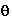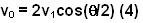## Sunday, June 1, 2008

### Irodov Problem 1.169Since the collision is perfectly elastic and there are no external forces acting on the system both momentum and energy will be conserved. As seen from the figures we have,From squaring (1) and equating to (2) we have,.
b)As in part a), the energy and momentum of the system must be conserved since it is an elastic collision and there are no external forces. Since the balls fly of symmetrically after collision with an angle of divergence ofthey move at an anglewith respect to the original direction of mass m1 as shown in the figure. Hence we have,From (1) and (2) we have,From (4) and (3) we have,